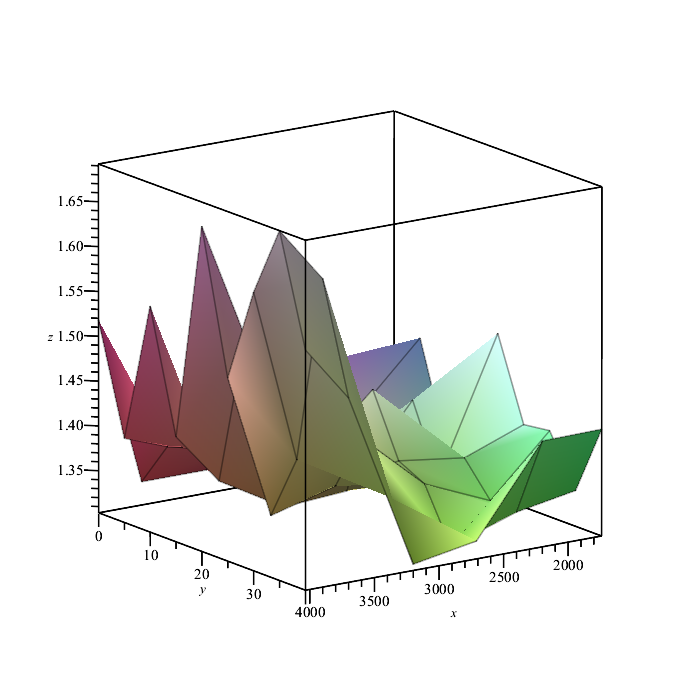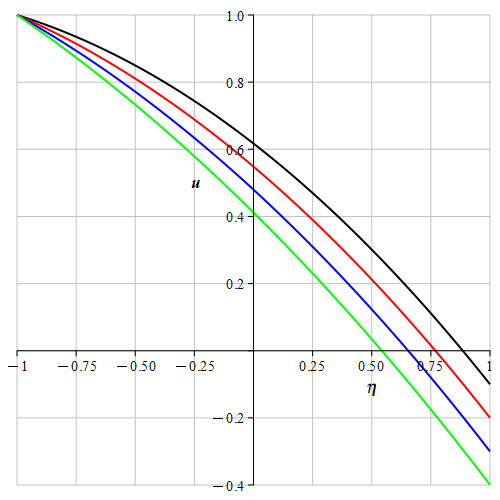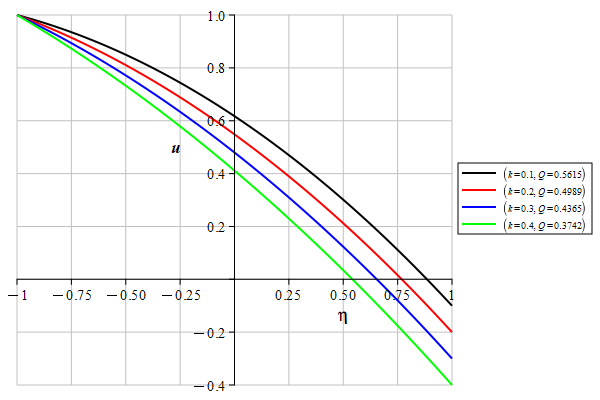Carl Love

## 23980 Reputation

9 years, 313 days
Natick, Massachusetts, United States
My name was formerly Carl Devore.

## indets...

If p is the PLOT structure, then do

rhs~(indets(p, "originalview"=anything))[];
[-0.986250000000000071 .. 9.98625000000000007, -1. .. 1.]

Using "magic" position numbers and op seems risky: The numbers could easily change. Using getdata is slightly more robust, but still involves magic numbers. My method is based on the keyword "originalview"; it uses no magic numbers.

Edit: The identical(...that I had before is superfluous: A string constant can be used itself as a type.

## Posts vs Questions...

I recently wrote a lengthy description of the distinction between Posts and Questions. It is the second Comment attached to this Post. The vast majority of threads are classified as Questions. For example, clicking on "Active Conversations" shows all threads in reverse chronological order by most-recent update, 50 per page. At the moment the first page shows 44 Questions and 6 Posts.

Regarding the phrase "posting a question": Yes, in common English, one definition of the verb to post is to place written material where it can be read by the general public. This usage is hundreds of years old. English has several times as many nouns as verbs. The definitions of the verbs gradually become more general than the nouns (if any) that they were originally associated with. (A post is a long piece of wood driven into the ground. Attaching written material to a post thus became posting it.)

It's not clear to me whether you just want to add the numeric entries, or if you want to do something else as well. If you just want to add the numeric entries, then all that you need to do is

The entries that haven't been defined will simply be ignored; there's no need for you to sort or remove or account for them in any way.

I strongly recommend that in the future, you use Array, not array, which has been obsolete for nearly 20 years.

## solve, log10...

Q1: To get all 3 complex solutions, you can do

solve(c^3 = 1000)

To restrict solutions to the real case, do

solve({c^3 = 1000, c > 0})

Q2: The command for a base-10 logarithm is log10(...). The command for a base-b logarithm is log[b](...), and that can also be used when b=10.

Q3: The inverse function of y=log[b](xis x=b^y.

## nprintf and MathML...

I don't think that it's possible to get color-enriched text with printf (not sure about that). But it can be done with nprintf (which has identical syntax to printf) like this:

```#With the improvements suggested by acer:
Vprint:= (v::{string, symbol}, k)->
nprintf('`#mrow(mn("%s]", mathcolor= "green"), mn(" is %a"));`', v, k)
:
Vprint(`1:1`, 4);
```

The operators inside the string (mrowmn,  mo, etc.) are not documented in Maple's help. They're from a language called MathML for which there are numerous online help pages. For example, Google search "MathML mrow" (without the quotes). (There are some MathML features that don't work in Maple.)

The green can be replaced by any 6-digit hexadecimal RGB (Red Green Blue) code preceded by #; for example, #00A000 is a medium green.

The same MathML operators (mrowmn, etc.) can be accessed from the Typesetting package, as shown by acer. Whether you do it with Typesetting or nprintf, Maple's Standard GUI display will prettyprint them the same. But Maple's kernel will treat them differently: The output of nprintf is a symbol (in the specific Maple sense of that word), and it can be used as a vertex label in a Graph; but the output of Typesetting is a function (in the specific Maple sense of that word), and it cannot be used in a Graph. If you're only interested in the prettyprinted display, acer's Typesetting method is easier to code in a procedure.

## Recursive assignment...

This line is the most-proximate cause of your errors:

i := unapply(add(i[k](t)*p^k, k = 0 .. n), t);

Now, I only spent about 1 minute looking at your code. There's certainly something wrong with that line, but I don't want to say (yet) that it's completely wrong---perhaps there is some valid concept that you were trying to express. But note that your equivalent lines s:= ..., e:= ..., and r:= ... do not use the same variable on the left and right sides of the assignment.

You may think that and x[k] are distinct independent variables---they are not. Assignments made to either will affect the other. But they aren't the same variable either! They are neither completely the same nor completely different. Their situation is somewhat akin to quantum entanglement but asymmetric---the x[k] being a completely dependent "child" of its "parent" x: Erasing also erases x[k], but erasing x[k] doesn't erase x.

There is a commonly given workaround for this situation, but it doesn't apply in this case. It's to use x__k instead of x[k]. The variables x and x__k are distinct and independent. This workaround doesn't work in this case because k and x__k are also independent.

And there's also a less-mysterious commonly given explanation of the connection between x and x[k] which also doesn't apply in this case: x is a table (akin to an array) and x[k] is an entry (element) of that table. This explanation doesn't apply when x is a procedure, which is what the result of unapply is. In that case, the invocations x(...and x[...](...invoke exactly the same procedure. The procedure can detect that it was invoked with the extra [...] if it chooses to check that; but if it doesn't check, then the two invocations do exactly the same thing, which is what is happening in your case.

In other words, in your line

i:= unapply(add(i[k](t)*p^k, k = 0 .. n), t)

the [k] is ultimately ignored because the line makes i into a procedure that doesn't check whether it was invoked with an index (such as ) attached. That doesn't happen with your other similar lines such as

s:= unapply(add(g[k](t)*p^k, k = 0 .. n), t)

because g is just an unassigned variable, not a procedure.

## `%+`(sort(..., key= sign*content)[])...

Maple has a very persistent memory of expressions that it's "seen before" in what it considers to be a mathematically equivalent form within the current session (regardless of whether they've been displayed). For `+` expressions, changing the order of terms is considered an equivalent form. This memory is formally called the simplification table, and it is fundamental to Maple's efficiency. This table is the reason that Maple seems to be ignoring your attempt to turn the list back into a polynomial. There are some ways to get Maple to forget that an expression is in the simplification table, but it can be very frustrating and not worth the effort.

As you said, you could use a list. An inert `%+` expression will work also, and that's almost equivalent to a list: a single "root" (or 0th) operator with a one-dimensional sequence of operands.

It can be done like this:

pn:= (a + sqrt(2)*a - a + a)^5:
`%+`(sort([op](expand(pn)), key= (sign*content)@evalf)[]);

I threw in that sqrt(2) and minus sign to show by example that the evalf and sign are needed.

## PLOT3D(MESH(Array(...)))...

If your request labelled (1), the curves, is merely a step to get to step (2), the surface, then there's no point in getting the curves, because getting the surface alone is substantially easier. It can be done like this:

Step 1: Change all the equations to assignment statements, i.e., X= ... should be X:= ..., F(X,5)= ... should be F(X,5):= ..., etc.

Step 2 (optional): Suppress the output of all these assignments by ending those statements with colons. It should be suppressed because it's redundant, it's ugly, and it bloats the GUI memory eventually making the worksheet to become unbearably sluggish to work with.

Step 3:

A:= Array(
(1..numelems(X), 1..numelems(Y), 1..3),
(i,j,k)-> `if`(k=1, X[i], `if`(k=2, Y[j], F(X, Y[j])[i])),
datatype= hfloat
):
plots:-display(PLOT3D(MESH(A)), labels= [x,y,z]);## remove...

While solve is capable of algebraically[*1] solving equations containing derivatives, I agree that it's not a good choice for this situation, and there's a much simpler and more-efficient method. And I can even make my method work in the context of the simplify command, like this:

```restart:

`simplify/Zero`:= proc(e)
local One;
remove(t-> is(t<>0), One*e) assuming One > 0
end proc:

simplify(a*f(x)*diff(f(x),x), Zero) assuming a>0, diff(f(x),x) > 0;
f(x)

```

[*1] solve doesn't solve differential equations per se, but it's capable of treating derivatives as if they were simple variables and isolating them algebraically to one side of an equation.

## member...

My algorithm to do this will work for any lists, so I wrote two tiny procedures for this. The first, WhereIn, is for lists A and B; the second, WhereInMatrix, converts the matrices to listlist form and then calls the first. The output of either is a set of equations i=j such that the ith element or row of A equals the jth element or row of B.

If an element of A is equal to multiple elements of B, only one such element of is given in the output. I can easily change this aspect if you want.

This code (like almost all my code) must be run in 1D input (Maple Input), not the default 2D Input.

```WhereIn:= (A::list, B::list)->
local i,j,a; {for i,a in A do if member(a, B, 'j') then i=j fi od}
:
WhereInMatrix:= (A::Matrix, B::Matrix)-> WhereIn(convert~([A,B], 'listlist')[])
:
(C1,C2):= 'combinat:-randcomb([\$9],4)'\$2;
C1, C2 := [6, 7, 8, 9], [1, 2, 6, 9]

(A,B):= 'LinearAlgebra:-RandomMatrix(9,2)'\$2:
WhereInMatrix(A,B);
{}
A[C1]:= B[C2]:
WhereInMatrix(A, B);
{6 = 1, 7 = 2, 8 = 6, 9 = 9}

```

## normal...

I don't know why you think combine is supposed to be equivalent to Mathematica's Together. Reading the help page for Together, to me it seems equivalent to Maple's normal. On the other hand, using combine and Together on sin(x)*cos(x) shows that those commands are quite different. (I tested Together[sin(x)*cos(x)] on WolframAlpha.com because I don't have Mathematica.)

But I'll agree with you that your example seems to violate the first sentence of the help page ?combine:

• The combine function applies transformations which combine terms in sums, products, and powers into a single term.

## Procedure for it...

Here's Maple code for your algorithm. Please test it thoroughly; I've only tested it superficially.

 > restart:
 > vs140580_algo:= proc(G::Graph, k::posint) uses GT= GraphTheory, It= Iterator; local     V:= subs(_V= GT:-Vertices(G), k-> _V[k]),     n:= GT:-NumberOfVertices(G),     A:= op(4,G), #neighbor array     L:= table(), #indices are the "labelled" vertices     LS, S, s, v, none_new,     R:= table() #output ;     for s in It:-Combination(n,k) do         S:= 1+~{seq}(s);         L:= table([S[], seq(A[v][], v= S)]=~ ());         do            none_new:= true;            LS:= {indices}(L, 'nolist');            if nops(LS)=n then R[V~(S)]:= (); break fi;            for v in {seq}(A[v][], v= LS) minus LS do                if A[v] subset LS then                    none_new:= false;                    L[v]:= ()                fi            od         until none_new     od;     {indices}(R, 'nolist') end proc :
 > GT:= GraphTheory: RG:= GT:-RandomGraphs:
 > G:= RG:-RandomGraph(10,.5);> GT:-DrawGraph(G);> vs140580_algo(G,2);> vs140580_algo(G,5);> nops(%), binomial(10,5);>

## selectremove...

All that you need is

(L_string, L_other):= selectremove(type, L, string)

## Don't transpose...

Your Pts is a list of 2-element row vectors. Apparently, pointplot doesn't like that they're row vectors, although its help page doesn't make that clear; it just says "Vector containing two numbers" without specifying an orientation. If you get rid of all the Transpose commands, then they'll be column vectors, and everything will work.

## A few easy changes...

I'm not sure exactly what you mean by "according to required desire" (which sounds weird in English), so I'm going to assume that you mean "aesthetically".

I'd do the following, which I've listed in decreasing order of aesthetic importance (which is of course a matter of personal judgement):

1. Change the gridlines color from black to gray.
2. Change the gridlines thickness from to (which isn't as small as it sounds).
3. Add thickness= 2 to the main part of the plot.
4. Change the labelfont to ["TIMES", "BOLDITALIC", 13]. The u in Helvetica looks weird to me because its descender (bottom right corner) is barely visible.

With just those very easy changes, I get this:With a bit more effort, a legend can be added:

```kQs:= k=~ .1*[\$4], Q=~ [.5615, .4989, .4365, .3742]:
plot(
subs~(kQs, M= .2, N= 2, x= 0, u), eta= -1..1,
color= [black, red, blue, green],
legend= ``~(kQs), legendstyle= [location= right, font= ["TIMES", 8]],
thickness= 2,
labels= ['eta', 'u'], labelfont= ["TIMES", "BOLD", 13], size= 200*[3,2],
axis= [gridlines= [8, thickness= 0, color= gray]]
);
```﻿